﻿ 非均匀二维蜂巢晶格中手征性d + id超导态的量子蒙特卡罗探究 Quantum Monte Carlo Study of the Chiral d + id Superconducting State on the Inhomogeneous Two-Dimensional Honeycomb Lattice

Vol.06 No.02(2017), Article ID:20777,11 pages
10.12677/CMP.2017.62003

Quantum Monte Carlo Study of the Chiral d + id Superconducting State on the Inhomogeneous Two-Dimensional Honeycomb Lattice

Shichao Fang1, Yun Gao2, Zhongbing Huang1*

1Faculty of Physics and Electronic Technology, Hubei University, Wuhan Hubei

2School of Materials Science and Engineering, Hubei University, Wuhan Hubei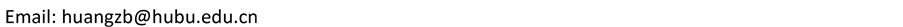Received: May 5th, 2017; accepted: May 24th, 2017; published: May 27th, 2017ABSTRACT

On the basis of the Hubbard model, the evolution of the chiral d + id superconducting state with the inhomogeneity on the two-dimensional honeycomb lattice is studied by using the constrained-path Monte Carlo method. We find that when the on-site U is small, the d + id pairing correlation function firstly increases with increasing the inhomogeneity, and then decreases beyond a certain inhomogeneity. For relatively larger U, the enhancement tendency of d + id pairing correlation function is strongly suppressed. Our results indicate that there exists an optimal inhomogeneity at small U values, which optimizes the d + id superconducting state on the honeycomb lattice. Further analysis shows that magnetism is not responsible for the inhomogeneity-dependent superconductivity. In terms of the changes of the density of states at the Fermi level and the effective on-site interaction as a function of inhomogeneity, we offer a reasonable explanation for the inhomogeneity-dependent superconductivity. Our study provides an important route for controlling the superconducting state on the honeycomb lattice.

Keywords:d + id Superconductivity, Chiral, Honeycomb Lattice, Quantum Monte Carlo

1湖北大学物理与电子科学学院，湖北 武汉

2湖北大学材料科学与工程学院，湖北 武汉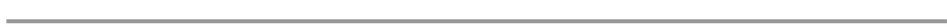.

1. 引言

2. 模型结构和哈密顿量(1)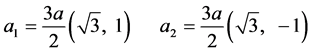(2)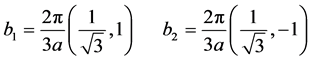(3)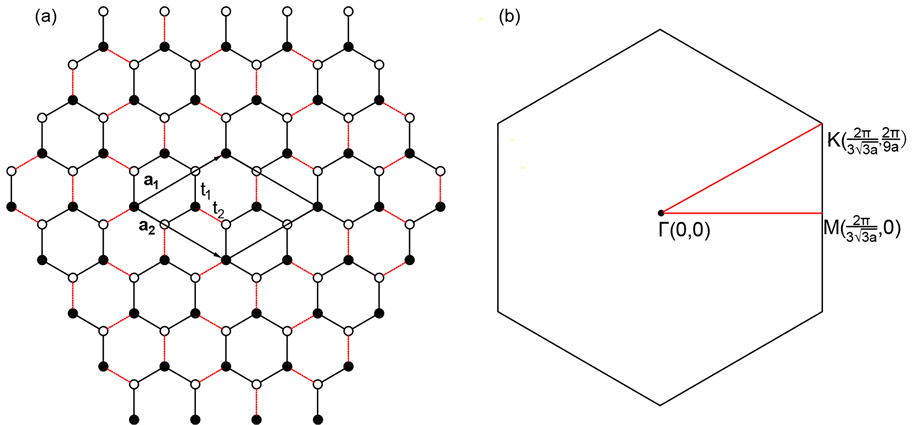Figure 1. (a) Schematic diagram of the inhomogeneous honeycomb lattice with double-48 sites; (b) Sketch of the first Brillouin zone. In the left panel, filled and open circles represent the A and B sub-lattice sites, a1 and a2 denote the basis vectors of the unit cell, and t1 and t2 stand for the hopping integrals within and between honeycombs(4)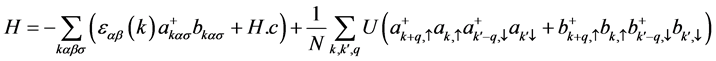(5)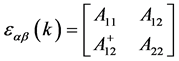(6)(7)(8)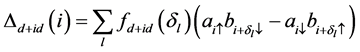(9)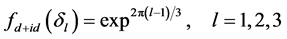(10)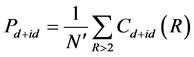(11)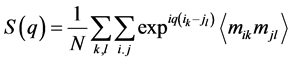(12)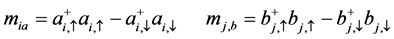(13)

3. 计算结果与讨论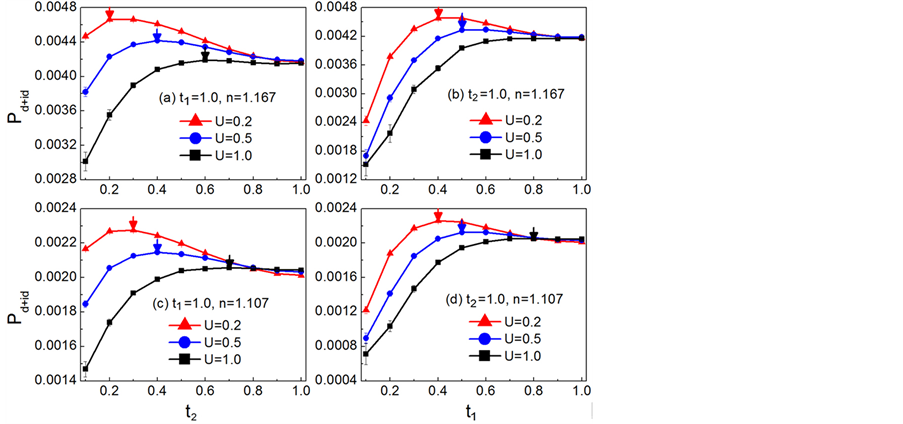Figure 2.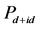as a function of the inhomogeneity for different lattice sites and on-site Hubbard interactions. (a) The size of lattice is 2 × 48, n = 1.167and t1 = 1.0; (b) The size of lattice is 2 × 48, n = 1.167 and t2 = 1.0; (c) The size of lattice is 2 × 75, n = 1.107 and t1 = 1.0; (d) The size of lattice is 2 × 75, n = 1.107 and t2 = 1.0Figure 3. Pairng correlation function Cd + id(R) of the chiral d + id superconducting state as a function of distance for different hopping integral t2 or t1 on the 2 × 108 lattice and n = 1.130. (a) t1 = 1.0, U = 0.2; (b) t2 = 1.0, U = 0.2; (c) t1 = 1.0, U = 0.5; (d) t2 = 1.0, U = 0.5; (e) t1 = 1.0, U = 1.0; (f) t2 = 1.0, U = 1.0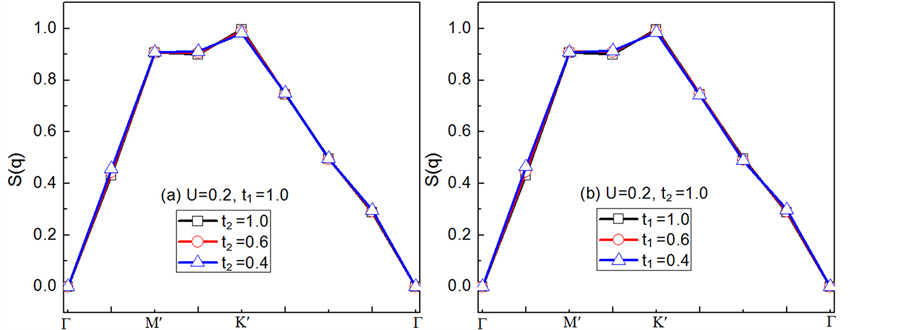Figure 4. Spin correlation S(q) on the 2 × 48 lattice along the high symmetric lines in the first Brillouin zone at n = 1.167 and U = 0.2. (a) t1 = 1.0; (b) t2 = 1.0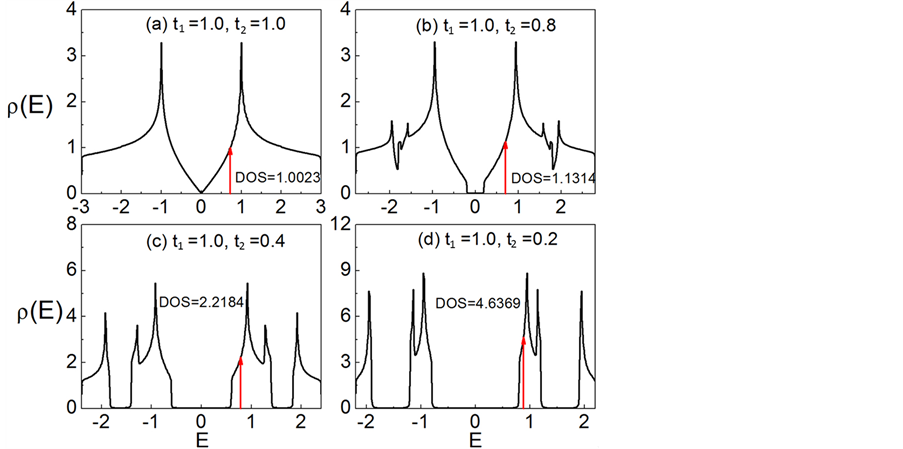Figure 5. Density of states for different t2 at the fixed t1 = 1.0. (a)-(d) correspond to t2 = 1.0, 0.8, 0.4, and 0.2, respectively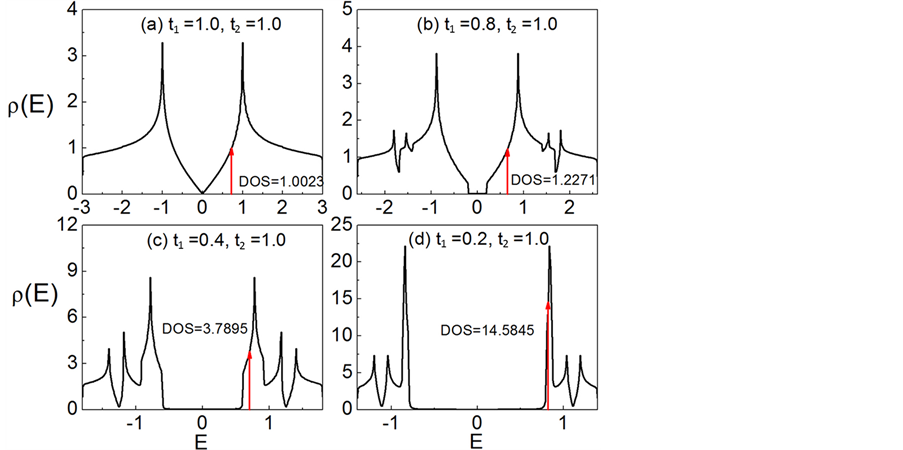Figure 6. Density of states for different t1 at the fixed t2 = 1.0. (a)-(d) correspond to t1 = 1.0, 0.8, 0.4, and 0.2, respectively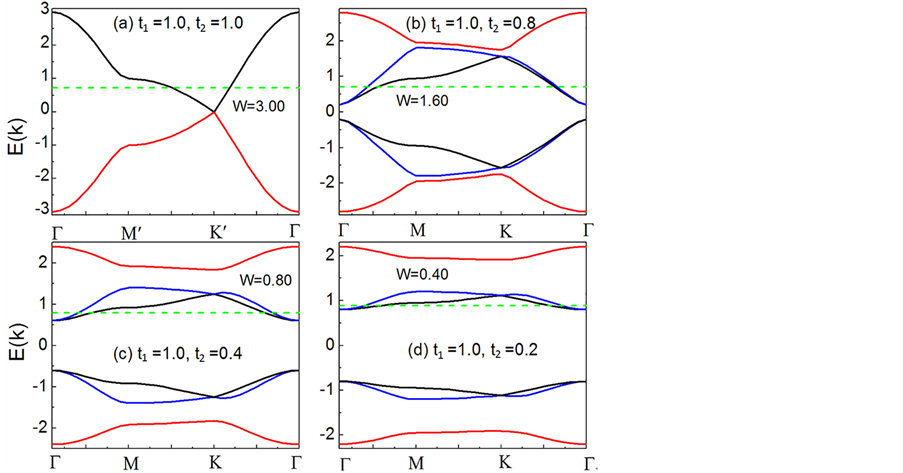Figure 7. Energy dispersion along the high symmetric lines for different t2 at the fixed t1 = 1.0. (a)-(d) t2 = 1.0, 0.8, 0.4, and 0.2, respectively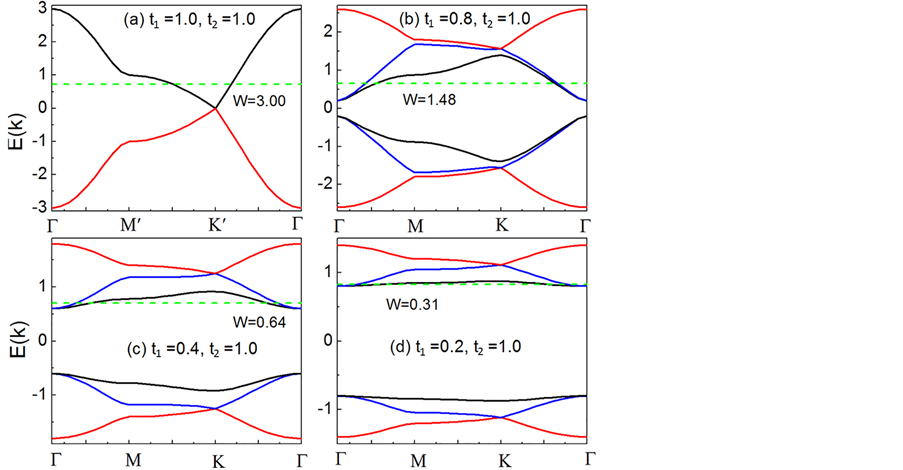Figure 8. Energy dispersion along the high symmetric lines for different t1 at the fixed t2 = 1.0. (a)-(d) t1 = 1.0, 0.8, 0.4, and 0.2, respectively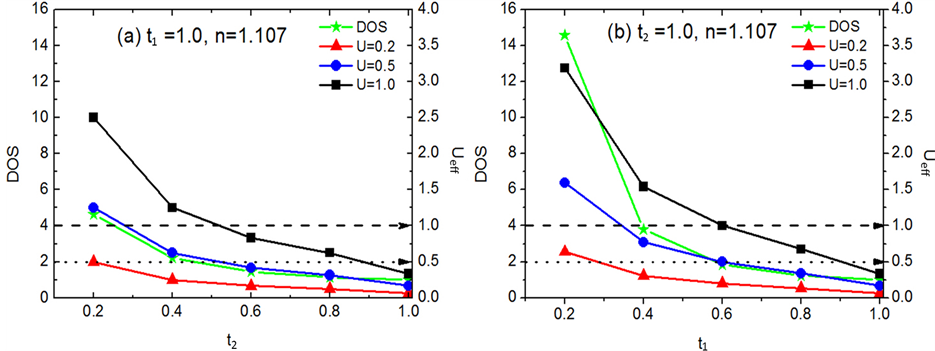Figure 9. Density of states at the Fermi level and effective on site Hubbard interaction Ueff as a function of t1 or t2 at n = 1.107. (a) and (b) correspond to t1 = 1.0 and t2 = 1.0, respectively

4. 结论

Quantum Monte Carlo Study of the Chiral d + id Superconducting State on the Inhomogeneous Two-Dimensional Honeycomb Lattice[J]. 凝聚态物理学进展, 2017, 06(02): 16-26. http://dx.doi.org/10.12677/CMP.2017.62003

1. 1. Nandkishore, R., Levitov, L.S. and Chubukov, A.V. (2012) Chiral Superconductivity from Repulsive Interaction in Doped Graphene. Nature Physics, 8, 158-163. https://doi.org/10.1038/nphys2208

2. 2. Pathak, S., Shenoy, V.B. and Baskaran, G. (2010）Possilble High-Temperature Superconducting State with a d+id Pairing Symmetry in Doped Graphene. Physical Review B, 81, Article ID: 085431. https://doi.org/10.1103/PhysRevB.81.085431

3. 3. Black-Schaffer, A.M., Wu, W. and Hur, K.L. (2014) Chiral D-Wave Super-conductivity on the Honeycomb Lattice Close to the Mott State. Physical Review B, 90, Article ID: 054521. https://doi.org/10.1103/PhysRevB.90.054521

4. 4. Shih, C.T., Lee, T.K., Eder, R., Mou, C.Y. and Chen, Y.C. (2004) Enhance-ment of Pairing Correlation by t’ in the Two-Dimensional Extended t-J Model. Physical Review Letters, 92, Article ID: 227002. https://doi.org/10.1103/PhysRevLett.92.227002

5. 5. Prelovsek, P. and Ramsk, A. (2005) Spin-Fluctuation Mechanism of Su-perconductivity in Cuprates. Physical Review B, 72, Article ID: 012510. https://doi.org/10.1103/physrevb.72.012510

6. 6. Veilleux, A.F., Dare, A.M., Chen, L., Vilk, Y.M. and Tremblay, A.M.S. (1995) Magnetic and Pair Correlations of the Hubbard Model with Next-Nearest-Neighbor Hopping. Physical Review B, 52, Article ID: 16255. https://doi.org/10.1103/physrevb.52.16255

7. 7. Chen, K.S., Meng, Z.Y., Yang, S.X., Pruschke, T., Moreno, J. and Jarrell, M. (2013) Evolution of the Superconductivity in the Two-Dimensional Hubbard Model. Physical Review B, 88, Article ID: 245110. https://doi.org/10.1103/physrevb.88.245110

8. 8. Tsai, W.F., and Kivelson, S.A. (2006) Superconductivity in Inhomogeneous Hubbard Model. Physical Review B, 73, Article ID: 214510. https://doi.org/10.1103/physrevb.73.214510

9. 9. Tsai, W.F., et al. (2008) Optimal Inhomogeneity for Superconductivity: Finite-Size Studies. Physical Review B, 77, Article ID: 214502. https://doi.org/10.1103/physrevb.77.214502

10. 10. Baruch, S., and Orgad, D. (2010) Contractor-Renormalization Study of Hub-bard Plaquette Clusters. Physical Review B, 82, Article ID: 134537. https://doi.org/10.1103/physrevb.82.134537

11. 11. Ying, T. et al. (2014) Determinant Quantum Monte Carlo Study of D-Wave Pairing in the Plaquette Hubbard Hamiltonian. Physical Review B, 90, Article ID: 075121.

12. 12. Huang, Z.B., Lin, H.Q. and Gubernatis, J.E. (2001) Quantum Monte Carlo study of Spin, Charge, and Pair-ing Correlations in the t-t’-U Hubbard Model. Physical Review B, 64, Article ID: 205101. https://doi.org/10.1103/PhysRevB.64.205101

13. 13. Huang, Z.B., Lin, H.Q. and Gubernatis, J.E. (2001) Pairing, Charge, and Spin Correlations in the Three-Band Hubbard Model. Physical Review B, 63, Article ID: 115112. https://doi.org/10.1103/physrevb.63.115112

14. 14. Zhang, S.W., Carlson, J. and Gubernatis, J.E. (1997) Constrained Path Quan-tum Monte Carlo Method for Fermion Ground States. Physical Review B, 55, Article ID: 7464. https://doi.org/10.1103/PhysRevB.55.7464

15. 15. Zhang, S.W., Carlson, J. and Gubernatis, J.E. (1997) Pairing Correlations in the Two-Dimensional Hubbard Model. Physical Review Letters, 78, Article ID: 4486. https://doi.org/10.1103/physrevlett.78.4486

16. 16. Ma, T.X., Huang, Z.B., Hu, F.M. and Lin, H.Q. (2011) Pairing in Graphene: A Quantum Monte Carlo Study. Physical Review B, 84, Article ID: 121410(R). https://doi.org/10.1103/PhysRevB.84.121410